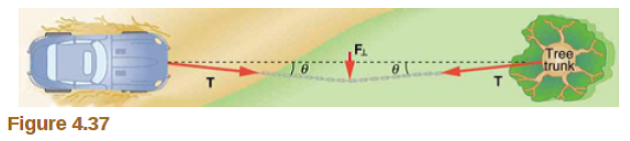# Problem: Suppose your car was mired deeply in the mud and you wanted to use the method illustrated in Figure 4.37 to pull it out. (a) What force would you have to exert perpendicular to the center of the rope to produce a force 12,000 N on the car if the angle is 2.00°? In this part, explicitly show how you follow the steps in the Problem-Solving Strategy for Newton's law of motion. (b) Real ropes stretch under such forces. What force would be exerted on the car if the angle increases 7.00° and you still apply the force found in part (a) to its center?

###### FREE Expert Solution

When a perpendicular force is applied on the rope, a tension is created in the rope.

The perpendicular force is balanced by the vertical component of the tension (vertical with respect to the image), which is induced in the rope.

Force in the vertical direction:

$\overline{){\mathbf{F}}{\mathbf{=}}{\mathbf{2}}{\mathbf{T}}{\mathbf{s}}{\mathbf{i}}{\mathbf{n}}{\mathbf{\theta }}}$, where T is the tension induced in the rope and θ is the angle of the rope from the ground.

89% (374 ratings)###### Problem Details

Suppose your car was mired deeply in the mud and you wanted to use the method illustrated in Figure 4.37 to pull it out. (a) What force would you have to exert perpendicular to the center of the rope to produce a force 12,000 N on the car if the angle is 2.00°? In this part, explicitly show how you follow the steps in the Problem-Solving Strategy for Newton's law of motion. (b) Real ropes stretch under such forces. What force would be exerted on the car if the angle increases 7.00° and you still apply the force found in part (a) to its center?